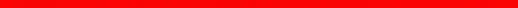Physics Department | Astronomy at UM |  Search

Review Checklist - Sample Questions

Laws of Motion and Gravity

Laws of Motion

• Why is Newton important in the history of astronomy?
• How does a body move if no force is acting on it?
• When two objects of different mass collide, is their motion equally affected?
• How does a rocket manage to lift off from the ground?
• Why does an airplane need an engine to keep flying, but the Moon stays up without one?
• When a ball falls toward the Earth because of gravity, does the Earth also feel attracted toward the ball?

Mass and Weight

• What is the difference between mass and weight?
• Are astronauts in an orbiting space shuttle subject to the Earth's gravity?
• What happens to the mass and weight of astronauts in space orbiting the Earth?
• Why do astronauts seem to float in space when orbiting the Earth?

Newton's Law of Gravity

• Is the weight of a baseball the same kind of force that attracts the Moon to the Earth?
• Is the Sun's motion affected by a gravitational pull from the Earth?
• How does the gravitational force between two bodies depend on their distance? On their masses?
• According to Newton's law of gravitation, which bodies exert a gravitational force on each other?
• What is the shape of the orbit of an object under the influence of the Sun's gravity?
• Is the shape of a planet's orbit really an ellipse?
• What allowed Newton to improve on Kepler's laws on planet motion?
• Can you apply Kepler's laws to the motion of a moon around a planet?
• Can an object feel the Sun's gravitational pull but not orbit in an ellipse around it?
• How can you find the mass of a distant star or a planet?
• What does the term escape velocity mean?

Consequences of Gravity

• What causes the tides along the coasts of Earth's oceans?
• Why does the Moon have a bigger effect than the Sun on the tides on Earth?page by luca bombelli <bombelli at olemiss.edu>, modified 29 sep 2012